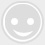# 详解单片机pwm控制基本原理

-回复 -浏览PWM是Pulse Width Modulation的缩写，它的中文名字是脉冲宽度调制，一种说法是它利用微处理器的数字输出来对模拟电路进行控制的一种有效的技术，其实就是使用数字信号达到一个模拟信号的效果。这是个什么概念呢？我们一步步来介绍。

#include

sbit PWMOUT = P0^0;

sbit ENLED = P1^4;

unsigned char HighRH = 0; //高电平重载值的高字节

unsigned char HighRL = 0; //高电平重载值的低字节

unsigned char LowRH = 0; //低电平重载值的高字节

unsigned char LowRL = 0; //低电平重载值的低字节

void ConfigPWM(unsigned int fr, unsigned char dc);

void ClosePWM();

void main(){

unsigned int i;

EA = 1; //开总中断

ENLED = 0; //使能独立 LED

while (1){

ConfigPWM(100, 10); //频率 100Hz，占空比 10%

for (i=0; i40000; i++);

ClosePWM();

ConfigPWM(100, 40); //频率 100Hz，占空比 40%

for (i=0; i40000; i++);

ClosePWM();

ConfigPWM(100, 90); //频率 100Hz，占空比 90%

for (i=0; i40000; i++);

ClosePWM(); //关闭 PWM，相当于占空比 100%

for (i=0; i40000; i++);

}

}

/* 配置并启动 PWM，fr-频率，dc-占空比 */

void ConfigPWM(unsigned int fr, unsigned char dc){

unsigned int high, low;

unsigned long tmp;

tmp = (11059200/12) / fr; //计算一个周期所需的计数值

high = (tmp*dc) / 100; //计算高电平所需的计数值

low = tmp - high; //计算低电平所需的计数值

high = 65536 - high + 12; //计算高电平的重载值并补偿中断延时

low = 65536 - low + 12;//计算低电平的重载值并补偿中断延时

HighRH = (unsigned char)(high>>8); //高电平重载值拆分为高低字节

HighRL = (unsigned char)high;

LowRH = (unsigned char)(low>>8); //低电平重载值拆分为高低字节

LowRL = (unsigned char)low;

TMOD &= 0xF0; //清零 T0 的控制位

TMOD |= 0x01; //配置 T0 为模式 1

TH0 = HighRH; //加载 T0 重载值

TL0 = HighRL;

ET0 = 1; //使能 T0 中断

TR0 = 1; //启动 T0

PWMOUT = 1; //输出高电平

}

/* 关闭 PWM */

void ClosePWM(){

TR0 = 0; //停止定时器

ET0 = 0; //禁止中断

PWMOUT = 1; //输出高电平

}

/* T0 中断服务函数，产生 PWM 输出 */

void InterruptTimer0() interrupt 1{

if (PWMOUT == 1){ //当前输出为高电平时，装载低电平值并输出低电平

TH0 = LowRH;

TL0 = LowRL;

PWMOUT = 0;

}else{ //当前输出为低电平时，装载高电平值并输出高电平

TH0 = HighRH;

TL0 = HighRL;

PWMOUT = 1;

}

}

#include

sbit PWMOUT = P0^0;

sbit ENLED = P1^4;

unsigned long PeriodCnt = 0; //PWM 周期计数值

unsigned char HighRH = 0; //高电平重载值的高字节

unsigned char HighRL = 0; //高电平重载值的低字节

unsigned char LowRH = 0; //低电平重载值的高字节

unsigned char LowRL = 0; //低电平重载值的低字节

unsigned char T1RH = 0; //T1 重载值的高字节

unsigned char T1RL = 0; //T1 重载值的低字节

void ConfigTimer1(unsigned int ms);

void ConfigPWM(unsigned int fr, unsigned char dc);

void main(){

EA = 1; //开总中断

ENLED = 0; //使能独立 LED

ConfigPWM(100, 10); //配置并启动 PWM

ConfigTimer1(50); //用 T1 定时调整占空比

while (1);

}

/* 配置并启动 T1，ms-定时时间 */

void ConfigTimer1(unsigned int ms){

unsigned long tmp; //临时变量

tmp = 11059200 / 12; //定时器计数频率

tmp = (tmp * ms) / 1000; //计算所需的计数值

tmp = 65536 - tmp; //计算定时器重载值

tmp = tmp + 12; //补偿中断响应延时造成的误差

T1RH = (unsigned char)(tmp>>8); //定时器重载值拆分为高低字节

T1RL = (unsigned char)tmp;

TMOD &= 0x0F; //清零 T1 的控制位

TMOD |= 0x10; //配置 T1 为模式 1

TH1 = T1RH; //加载 T1 重载值

TL1 = T1RL;

ET1 = 1; //使能 T1 中断

TR1 = 1; //启动 T1

}

/* 配置并启动 PWM，fr-频率，dc-占空比 */

void ConfigPWM(unsigned int fr, unsigned char dc){

unsigned int high, low;

PeriodCnt = (11059200/12) / fr; //计算一个周期所需的计数值

high = (PeriodCnt*dc) / 100; //计算高电平所需的计数值

low = PeriodCnt - high; //计算低电平所需的计数值

high = 65536 - high + 12; //计算高电平的定时器重载值并补偿中断延时

low = 65536 - low + 12; //计算低电平的定时器重载值并补偿中断延时

HighRH = (unsigned char)(high>>8); //高电平重载值拆分为高低字节

HighRL = (unsigned char)high;

LowRH = (unsigned char)(low>>8); //低电平重载值拆分为高低字节

LowRL = (unsigned char)low;

TMOD &= 0xF0; //清零 T0 的控制位

TMOD |= 0x01; //配置 T0 为模式 1

TH0 = HighRH; //加载 T0 重载值

TL0 = HighRL;

ET0 = 1; //使能 T0 中断

TR0 = 1; //启动 T0

PWMOUT = 1; //输出高电平

}

/* 占空比调整函数，频率不变只调整占空比 */

unsigned int high, low;

high = (PeriodCnt*dc) / 100; //计算高电平所需的计数值

low = PeriodCnt - high; //计算低电平所需的计数值

high = 65536 - high + 12; //计算高电平的定时器重载值并补偿中断延时

low = 65536 - low + 12; //计算低电平的定时器重载值并补偿中断延时

HighRH = (unsigned char)(high>>8); //高电平重载值拆分为高低字节

HighRL = (unsigned char)high;

LowRH = (unsigned char)(low>>8); //低电平重载值拆分为高低字节

LowRL = (unsigned char)low;

}

/* T0 中断服务函数，产生 PWM 输出 */

void InterruptTimer0() interrupt 1{

if (PWMOUT == 1){ //当前输出为高电平时，装载低电平值并输出低电平

TH0 = LowRH;

TL0 = LowRL;

PWMOUT = 0;

}else{ //当前输出为低电平时，装载高电平值并输出高电平

TH0 = HighRH;

TL0 = HighRL;

PWMOUT = 1;

}

}

/* T1 中断服务函数，定时动态调整占空比 */

void InterruptTimer1() interrupt 3{

static bit dir = 0;

static unsigned char index = 0;

unsigned char code table = { //占空比调整表

5, 18, 30, 41, 51, 60, 68, 75, 81, 86, 90, 93, 95

};

TH1 = T1RH; //重新加载 T1 重载值

TL1 = T1RL;

if (dir == 0){ //逐步增大占空比

index++;

if (index >= 12){

dir = 1;

}

}else{ //逐步减小占空比

index--;

if (index == 0){

dir = 0;

}

}

}